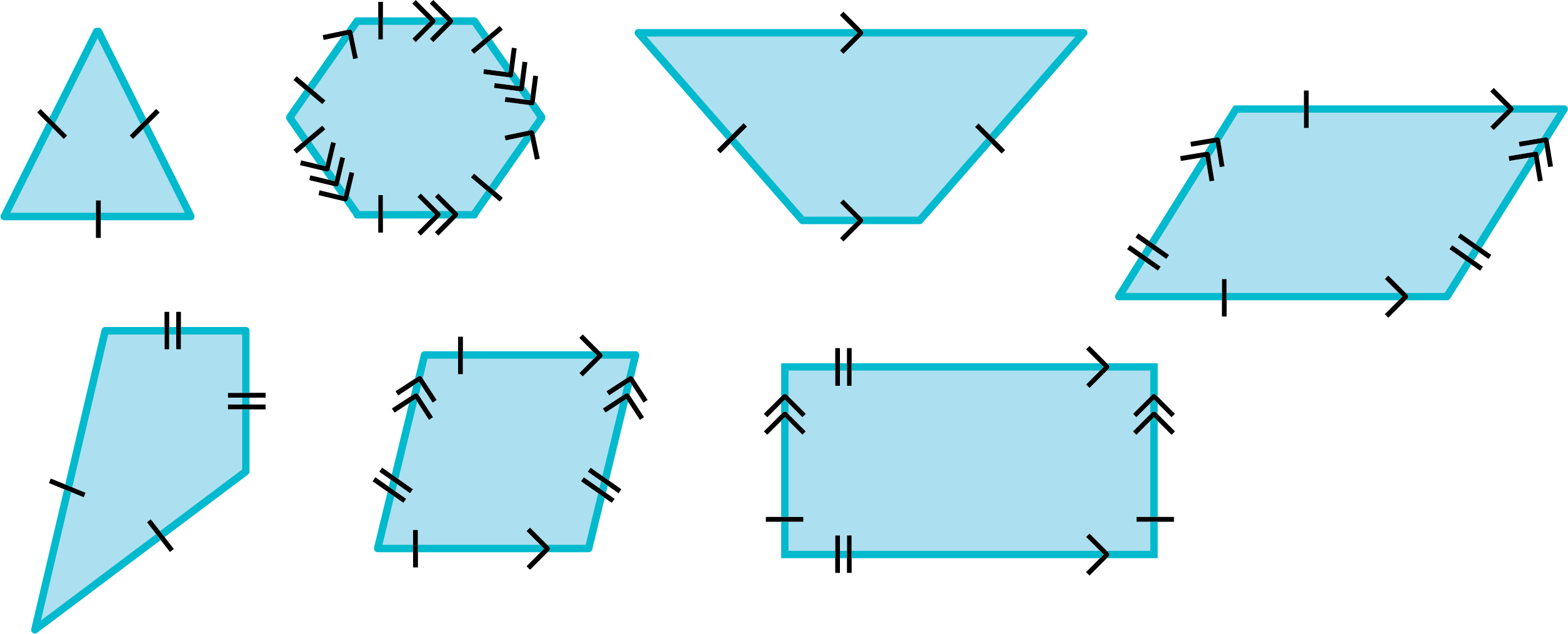### Home > CCG > Chapter 2 > Lesson 2.3.2 > Problem2-114

2-114.

Hannah’s shape bucket contains an equilateral triangle, an isosceles right triangle, a regular hexagon, a non-isosceles trapezoid, a rhombus, a kite, a parallelogram and a rectangle. If she reaches in and selects a shape at random, what is the probability that the shape will meet the criterion described below?

1. At least two sides congruent.

2. Two pairs of parallel sides.

3. At least one pair of parallel sides.

Draw the shapes and note which have congruent sides and parallel sides.$\large \frac{\text{Number of objects with desired characteristic}}{\text{Total number of objects}}$